# Multiplication and Division

Go back to  'Maths'

## The Big Idea: What are Multiplication and Division?

Multiplication is repeated addition. Division is repeated subtraction and can also be looked as breaking down a large group into several smaller, equal groups. That’s actually all there is to it. Simple right? Image A shows 4 groups of 3 apples each which is nothing but 4 x 3 = 12. It shows how multiplication can be visualised as repeated addition. Image B shows 14 fishes being equally moved into two different pots. Division as repeated subtraction can be seen here.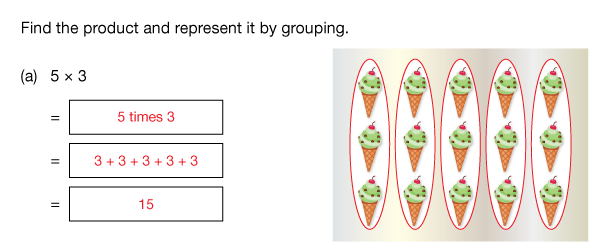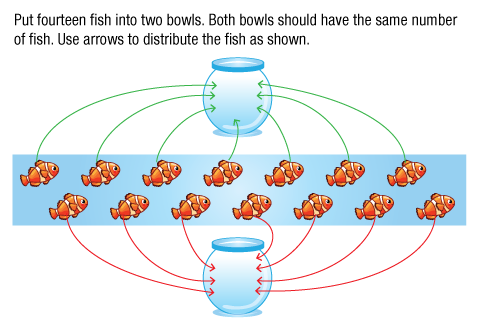## Topics closely related to Multiplication and Division

It is important, however, that, before multiplication and division are taught formally, children should be familiar with the following experiences and skills.

Children should:

1. Be able to count securely

2. Understand basic addition and subtraction

3. Be able to form groupings of the same size

Comfort with multiplying and dividing quantities can help in high school math and also math in our everyday lives.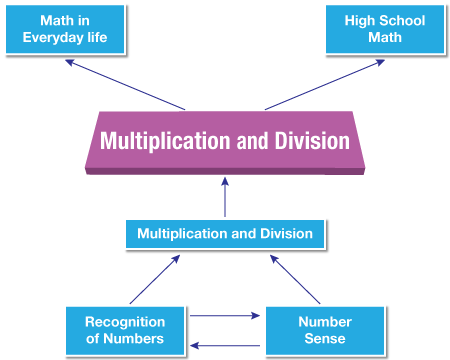## Flow of multiplication

Given below is the progression that should be adopted while teaching multiplication.

1. Moving from unitary counting to counting in multiples;

2. The notion of repeated addition (including number line modelling) and doubling;

3. Introduction of multiplication symbol.

4. Learning multiplication facts;

5. Simple scaling (by whole number scale factors) in the context of measurement or money;

6. Multiplication using standard algorithms ;

## Flow of Division

Given below is the progression that should be adopted while teaching Division.

1. The notion of ‘fairness’ and its application in equal sharing;

2. Refining equal sharing strategies;

3. Halving (including odd and even numbers as a by-product)

4. Division by repeated subtraction (including number line modelling);

5. Introduction of ÷ symbol and associated language;

6. Deriving division facts from multiplication facts (using division as the inverse of multiplication);

7. Informal written methods using repeated subtraction of the divisor;

8. Whole number remainders;

9. Dividing multiples of powers of 10 by powers of ten;

10. Informal written methods (using repeated subtraction of multiples of the divisor);

11. Expanded standard algorithm for short division;

12. Standard long division algorithm;

## How do you teach Multiplication and Division?

### 1. Use everyday objects to help children demonstrate multiplication & division.

2. Use Math manipulative such as colour counters to multiply and divide quantities.

3. Get a laminated sheet with multiplication facts written on it. You can ask children to represent the fact using some stickers or bindis.

4. Put charts at home. Every once in a while question the child related to something given on the chart.

## Misconceptions related to Multiplication & Division

1. Guide your child through the concept of place value. One of the most common mistakes that arise while multiplying two numbers of 2-digit or greater is that children forget to transfer the place value when they move to the second number. That zero is there for a reason, it’s because you have moved one place higher. From the ones place to the tens place, which necessitates the addition of the zero. In essence you are just decomposing the lower number and adding the sub-parts. The concept is clearly shown in the example below: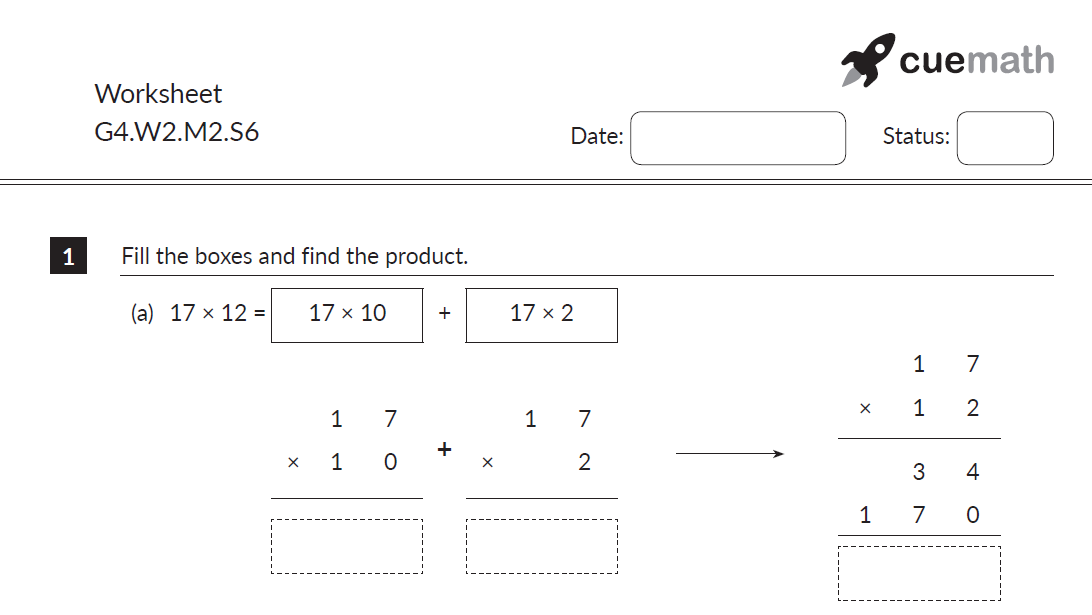2. A similar doubt occasionally arises when it comes to division. We’re talking about the situation when you are dividing two numbers and the divisor is greater than the part of the dividend being considered for that part of the operation. This usually leads to the appearance of a 0 in the quotient, and students, in their early stages with division struggle with this. The core concept at play here is that the Dividend = (Divisor x Quotient) + Remainder. So, if a zero arises in the quotient, it’s completely okay, and it’s not a mistake on the part of your child and urge them to carry on.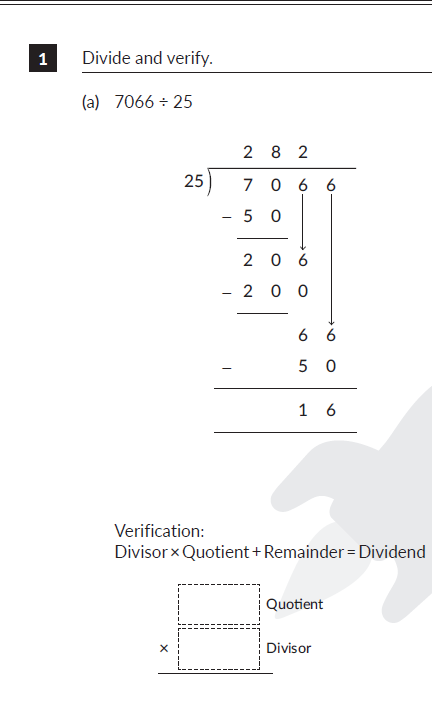Multiplication and Division
Multiplication and Division
Properties and Ideas